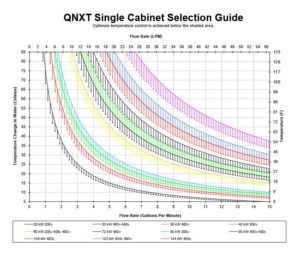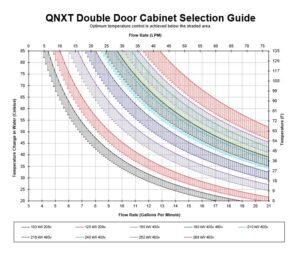# Deionized Water Heater Selection Guide

To determine which heater size is needed, calculate the required kilowatt with the following formula:

Required kW = 0.264*(Flow in GPM)*(Temp Delta in °C)

Conversion Calculations:
GPM = LPM / 3.8
°C = 5/9*(°F – 32)

Example
Ambient Water Temp = 25 °C
Desired Process Temp = 70 °C
Temperature Delta = 45 °C
Required kW = 0.264*(4 GPM)*(45 °C) = 47.5 kW

For optimal temperature response and to compensate for seasonal changes in ambient water temperature, we recommend adding 25% excess heating capacity.

47.5 kW(1.2) = 57 kW

The best option for this application is a 60kW heater.

###### Click the chart to enlarge